# Image transformation¶

## Translation¶

Translating an image is shifting it along the x and y axes. A affine transformation can be obtained by using a transformation matrix `M`. It is a translation matrix which shifts the image by the vector (x, y). The first row of the matrix is [1, 0, x], the second is [0, 1, y]

```M = np.float32([[1, 0, x], [0, 1, y]])
shifted = cv.warpAffine(img, M, size)
```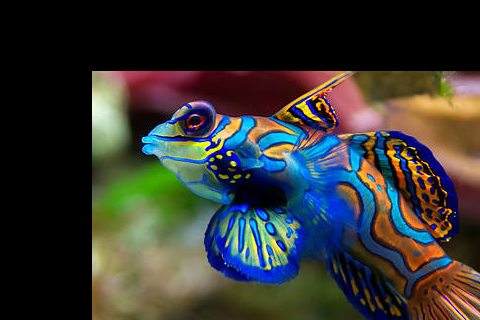`fish.jpg`

`transform1.py`

## Rotation¶

When we rotate an image we need to specify the center of rotation. Here we take the center of the image:

```h, w = img.shape[:2]
center = w//2, h//2
```

To obtain the rotation matrix we use the function `cv.getRotationMatrix2D`. It takes three arguments:

• the rotation center,
• the roation angle and
• the scale factor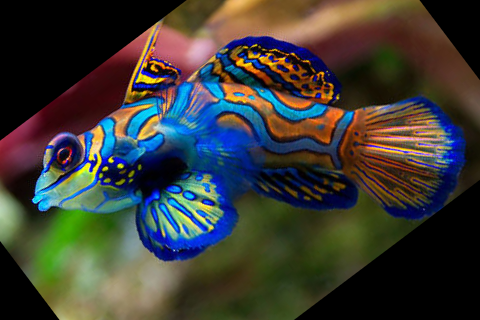```"""rotation an image using the trackbar."""
import cv2 as cv

def trackbar(angle):
M = cv.getRotationMatrix2D(center, angle, 1.0)
rotated = cv.warpAffine(img, M, (w, h))
cv.imshow('window', rotated)

h, w = img.shape[:2]
center = w//2, h//2

cv.imshow('window', img)
cv.createTrackbar('angle', 'window', 0, 180, trackbar)

cv.waitKey(0)
cv.destroyAllWindows()
```

`transform2.py`

## Scale¶

Scaling an image is to change its dimension.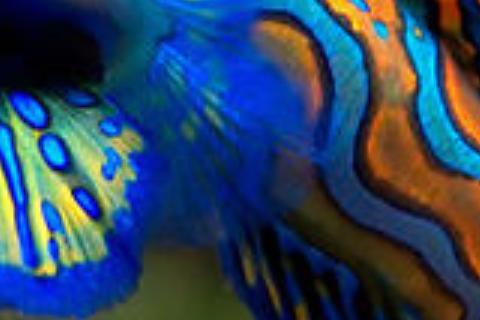```"""scale an image using the trackbar."""
import cv2 as cv

def trackbar(scale):
M = cv.getRotationMatrix2D(center, 0, scale/10)
rotated = cv.warpAffine(img, M, (w, h))
cv.imshow('window', rotated)

h, w = img.shape[:2]
center = w//2, h//2

cv.imshow('window', img)
cv.createTrackbar('scale', 'window', 10, 30, trackbar)

cv.waitKey(0)
cv.destroyAllWindows()
```

`transform3.py`

## Flipping¶

Horizontally or vertically using a key.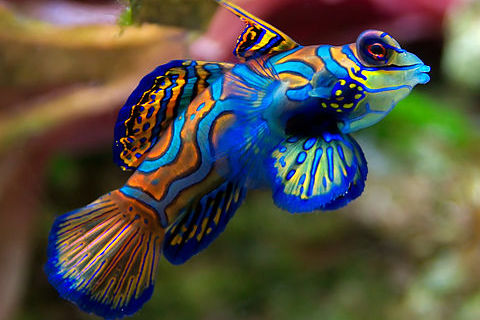```"""Flip an image horizontally and vertically using keys."""
import cv2 as cv

cv.imshow('window', img)

while True:
k = cv.waitKey(0)
if k == ord('q'):
break

elif k == ord('v'):
img = cv.flip(img, 0)

elif k == ord('h'):
img = cv.flip(img, 1)

cv.imshow('window', img)

cv.destroyAllWindows()
```

`transform4.py`

## Image artithmetic¶

The operation `add` and `subtract` allow to add two images. The `add` function is limited to 255. The `subtract` function is limit to 0. In the example below we add or subtract the value (40, 40, 40) to each pixel. As a result, the image becomes brighter or darker.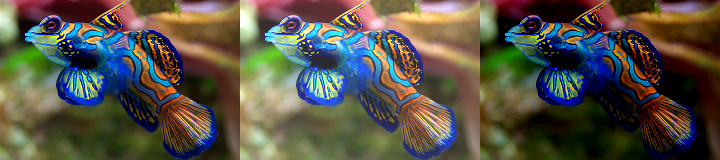```"""Add and subtract"""
import cv2 as cv
import numpy as np

img = cv.resize(img, None, fx=0.5, fy=0.5, interpolation=cv.INTER_CUBIC)
M = np.ones(img.shape, dtype='uint8') * 40

darker = cv.subtract(img, M)

img2 = np.hstack([img, brighter, darker])

cv.imshow('window', img2)
cv.waitKey(0)
cv.destroyAllWindows()
```

`transform5.py`

## Bitwise operations¶

Bitwise operations act on grayscale images. Most often it is used on black and white images. We start wtih a circle and a square shape and calculate this three bitwise operations:

• and
• or
• xor (exclusive or)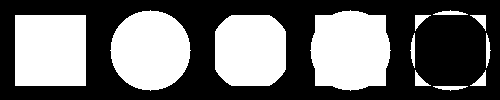```"""Bitwise and, or and xor operation"""
import cv2 as cv
import numpy as np

d = 15
rect = np.zeros((100, 100), np.uint8)
cv.rectangle(rect, (d, d), (100-d, 100-d), 255, -1)

circle = np.zeros((100, 100), np.uint8)
cv.circle(circle, (50, 50), 40, 255, -1)

bit_and = cv.bitwise_and(rect, circle)
bit_or = cv.bitwise_or(rect, circle)
bit_xor = cv.bitwise_xor(rect, circle)

img = np.hstack([rect, circle, bit_and, bit_or, bit_xor])

cv.imshow('window', img)
cv.waitKey(0)
cv.destroyAllWindows()
```

`bitwise.py`

We can use a mask to extract only a certain part of an image.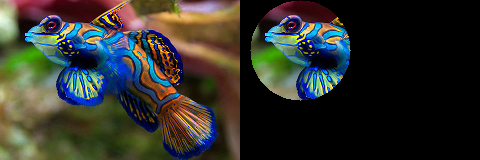```"""Masking."""
import cv2 as cv
import numpy as np

img = cv.resize(img, None, fx=0.5, fy=0.5, interpolation=cv.INTER_CUBIC)
cv.circle(mask, (60, 50), 50, 255, -1)

cv.imshow('window', img2)
cv.waitKey(0)
cv.destroyAllWindows()
```

`masking1.py`

## Splitting channels¶

We can split an RGB image into it’s components. Let’s use an image which contains the three base colors.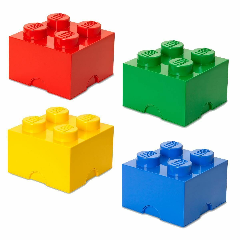`lego.png`

We find each color component in the separate channel Blue-Green-Red.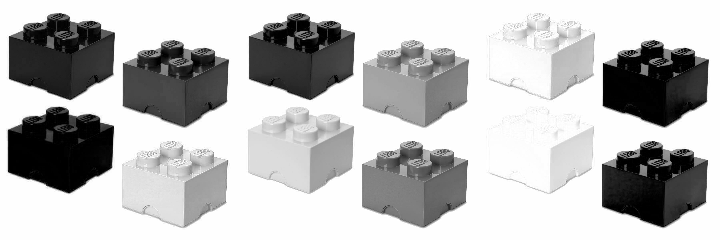```"""Splitting into 3 channels"""
import cv2 as cv
import numpy as np

b, g, r = cv.split(img)
img2 = np.hstack([b, g, r])

cv.imshow('window', img2)
cv.waitKey(0)
cv.destroyAllWindows()
```

`splitting1.py`

## Merging channels¶

We can merge channels.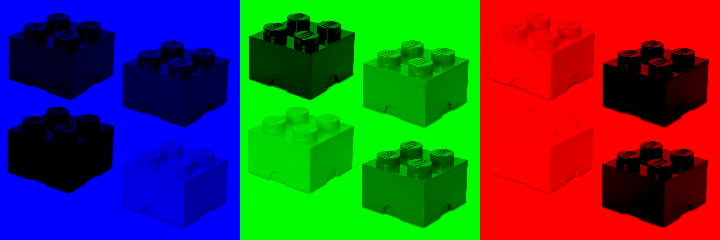```"""Merging 3 channels"""
import cv2 as cv
import numpy as np

z = np.zeros(img.shape[:2], 'uint8')

b, g, r = cv.split(img)
blue = cv.merge([b, z, z])
green = cv.merge([z, g, z])
red = cv.merge([z, z, r])

img2 = np.hstack([blue, green, red])

cv.imshow('window', img2)
cv.waitKey(0)
cv.destroyAllWindows()
```

`splitting2.py`

A different and faster way of keeping only one color channel and setting the others to zero is to act directly on the Numpy array using slice indexing.

```"""Numpy indexing."""
import cv2 as cv
import numpy as np

blue = img.copy()
green = img.copy()
red = img.copy()

blue[:, :, 1:] = 0
green[:, :, 0] = 0
green[:, :, 2] = 0
red[:, :, :2] = 0

img2 = np.hstack([blue, green, red])

cv.imshow('window', img2)
cv.waitKey(0)
cv.destroyAllWindows()
```

`splitting3.py`

## Color spaces¶

So far we have seen the RGB color space. However there are many other spaces.

The example below shows:

• HSV (Hue-Saturation-Value)
• L*a*b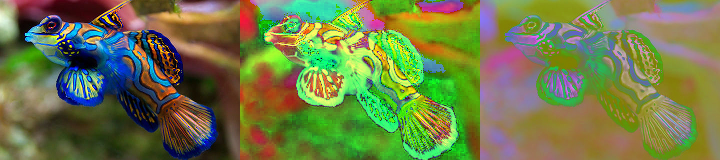```"""Change the color space."""
import cv2 as cv
import numpy as np

img = cv.resize(img, None, fx=0.5, fy=0.5, interpolation=cv.INTER_CUBIC)
M = np.ones(img.shape, dtype='uint8') * 40

hsv = cv.cvtColor(img, cv.COLOR_BGR2HSV)
lab = cv.cvtColor(img, cv.COLOR_BGR2LAB)
img2 = np.hstack([img, hsv, lab])

cv.imshow('window', img2)
cv.waitKey(0)
cv.destroyAllWindows()
```

`transform7.py`

## Affine transformation¶

Here we use the mouse to rotate and scale.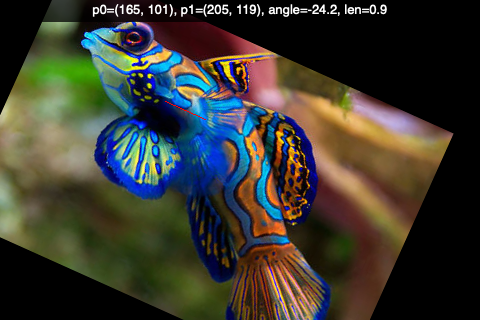```"""Rotate and scale image with mouse."""
import cv2 as cv
import numpy as np

RED = (0, 0, 255)
p0, p1 = (100, 30), (400, 90)

def mouse(event, x, y, flags, param):
global p0, p1

if event == cv.EVENT_LBUTTONDOWN:
p0 = x, y
p1 = x, y

elif event == cv.EVENT_MOUSEMOVE and flags == 1:
p1 = x, y

elif event == cv.EVENT_LBUTTONUP:
p1 = x, y

dx = p1 - p0
dy = p1 - p0
angle = -np.degrees(np.arctan2(dy, dx))
len = np.sqrt(dx**2 + dy**2) / 50
cv.displayOverlay('window', f'p0={p0}, p1={p1}, angle={angle:.1f}, len={len:.1f}')

M = cv.getRotationMatrix2D(p0, angle, len)
img2 = cv.warpAffine(img, M, (w, h))
cv.line(img2, p0, p1, RED, 2)
cv.imshow('window', img2)

`affine1.py`